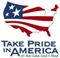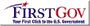Scientific Investigations Report 2006-5188

U.S. GEOLOGICAL SURVEY
Scientific Investigations Report 2006-5188

Estimated Trace-Metal Loads and Flow-Weighted Concentrations, 1991-2004

Regression models were developed and annual trace-metal loads and FWCs were estimated for Enaville (1992-2004), Pinehurst, Harrison, (1991-2004) and Post Falls (1991-2003) to understand the variability of metal loading with time. Because these load models and estimates cover a longer interval than the 1999-2004 study, they are described as “long-term.” Developing long-term regression models for these four sites also enabled a comparison of the load estimates produced for 1991-2004 with those for 1999-2004 and an examination of the robustness of the two differently calibrated models, specifically for the latter period. The metal concentration data for 1999–2004 were the same as those used for the short-term load simulation. The regression coefficients and coefficients of determination (R2) of the long-term models are presented in table 6. Long-term annual load estimates and FWCs are summarized in table 7. Graphs of annual estimated loads at all sites for all years are shown in figure 6. The graphs in figure 7 summarize the long-term mean annual metal loads for the four stations. The mean annual flow-weighted concentrations calculated from the long-term loads are shown in figure 8.

Long-term load estimates are similar to the results for 1999-2004 in terms of mean annual metal loads. For example, the largest mean loads for the estimation period were measured at Harrison and Pinehurst. Generally, the highest estimated loads were in 1996 and 1997 at all four stations, primarily because of extremely high flows. High loads also were measured in 1991. The lowest loads prior to 1999 generally were in lower-flow years, 1994 and 1998. Although low estimated loads in 1994 and 1998 probably reflect low streamflow, estimated loads in 1998 also may reflect a lack of sufficient time for metals to accumulate upstream after unusually high flows in 1996 and 1997 had scoured the channels.

Comparison with 1999-2004 Estimates

Long-term trace-metal load estimates for 1999-2004 generally compare well with the estimates produced by the short-term model for the overlapping time interval, but the similarity of the estimates varies from station to station, from year to year, and from metal to metal (table 8, fig. 9). The most consistent results for a single station were obtained from the gaging station at Pinehurst, where the long-term results for all metals are within 10 percent of the short-term estimates, except the TCd estimate for 2004. The estimated TZn load at Pinehurst is within 1 percent of the short-term estimates for all six years. Large differences in TCd load estimates were computed at Enaville in 1999 and in TPb estimates at Enaville, Harrison, and Post Falls for a number of years.

Because the load regression is based on the log-linear relation between flow and concentration, if the calibration data files represent a different range of flow or concentration values, the resulting regression may yield different load estimates due to the differences in regression line slopes. This is exemplified by the data for Pinehurst. The short-term load estimates for DPb are 17 to 54 percent lower than the long-term estimates. Comparison of the two calibration files shows the likely reason: the mean concentrations for most metals were in good agreement, except that the mean DPb concentration of the short-term calibration data was about 50 percent lower than that of the long-term data. This demonstrates the importance of assuring that input data adequately represent conditions during the time interval for which loads are estimated. Results should not be extrapolated beyond the time interval or flow regime represented by the input data.

Because the calibration data used in the long-term regressions and the short-term regressions were the same for 1999–2004, differences in the estimated loads for that period result from the differences in the regression equations produced by the model in the two cases. For example, the short- and long-term estimates for 2001 were similar at all four stations; the differences never exceeded 10 percent for any metal. This may be related to low streamflow (and consequently, load estimates) in 2001, the lowest in the 14-year estimation interval, a year that was common to both short- and long-term regressions. Conversely, the highest-flow years in the 14-year interval were 1996 and 1997, years that were not included in the short-term model regressions. The two models probably treat low-flow conditions similarly, but provide different results for high-flow conditions because calibration data for the long-term model provided data for low- and high-flow years, whereas data for the short-term model did not.

The value of long-term versus short-term load estimates ultimately depends on data available, questions being addressed, and purposes for which the results will be used. In this study, longer estimation intervals gave a more complete account of metal loading in the basin at the four long-term stations. Nevertheless, the most vital criterion to achieve the best results using LOADEST is that the calibration data be representative of the conditions, both hydrologic and temporal, during the simulated interval.

Temporal Trends in Long-Term Metal Load Estimates

To gain a better understanding of metal loading through time, the long-term annual trace metal load estimates were evaluated for possible temporal trends. LOADEST results include information on trends in the form of a statistically significant coefficient for a “time” term (labeled “f” in tables 2 and 6) in the regression equation. If present, a negative coefficient signifies an overall downward trend during the estimation period; a positive term indicates an upward trend. More complex changes with time are indicated by the presence of a statistically significant “time-squared” (t2) term in addition to a time term. In this study, a p-value of less than or equal to 0.05 (greater than or equal to the 95 percent confidence level) was considered statistically significant. According to the LOADEST results, all four long-term sites showed significant downward trends for DCd, TCd, DZn, and TPb. In addition, Enaville and Post Falls showed significant downward trends for DPb and TZn loads; Harrison TZn loads also decreased significantly with time. No gaging stations showed an increasing trend.

Metal loads and FWCs at Enaville generally decreased with time from 1992-2004, but some explanation is needed for the unusually smooth descending curve displayed by the annual mean FWCs of DCd (fig. 10), in which estimates ranged from 1.6 to 0.02 µg/L DCd. Measured concentrations of DCd were low at Enaville. Of the 86 concentration measurements used in the calibration file, 68 were censored data, for example, below the minimum detection limit (MDL). The 18 measured concentrations above the MDL ranged from 0.02 to 1.0 µg/L. Analytical precision improved during the estimation period and the MDLs changed from 1 to 0.04 µg/L in 2001. The LOADEST software is able to accommodate varying detection limits and applies statistical methods to enable use of censored data in the regression. The regular shape of the time-series graph of DCd FWC likely is an expression of combined effects of the high proportion of censored data for this constituent, modeling process itself, and actual decreases in FWC with time. A similar pattern is exhibited by DCd at Post Falls, another station with abundant censored calibration data. An example from Pinehurst of TCd FWCs calculated from data with no censored data among 77 samples is given for comparison (fig. 10).

A simple but useful independent test for trends in time-series data is the non-parametric Mann-Kendall trend test. Generally, this test determines whether values tend to monotonically increase or decrease with time. Because this test is non-parametric, the data need not be assumed to have a normal distribution. This is a valid test as long as no serial correlation is present in the data (Helsel and Hirsch, 1995). Because annual data were analyzed, there was no sensitivity to seasonal changes. The relatively long 13- or 14-year interval helps minimize the effects of extreme years.

The results of applying the Mann-Kendall trend test to estimated annual load and FWC data are compared with the trend information from the long-term LOADEST regression results in table 9.

Results of the two types of trend analysis agree in most cases. For example, both methods indicate that all four long-term sites had statistically significant (with 95 percent confidence) decreasing trends for Cd loads and FWCs for the estimation period, with a few cases of disagreement; for instance, the methods gave contradictory results for TZn at Pinehurst and at Post Falls.

It should be noted that the Mann-Kendall test results simply show whether an overall monotonic change exists for the entire 13- or 14-year period. Therefore, trends for shorter intervals in this period are not precluded. For example, based on the Mann-Kendall test, FWCs of DPb show a significant increasing trend for 1999-2003 at Post Falls. The statistical results must be viewed in the context of other information known about the data and about the system as a whole.U.S. Department of the Interior | U.S. Geological Survey